### Home > INT3 > Chapter 12 > Lesson 12.1.3 > Problem12-46

12-46.
1. Describe each transformation below in terms of the parent graph f(θ) = tan(θ). Homework Help ✎

1. g(θ) = tan(θ) + 1

2. h(θ) = tan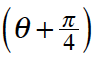3. j(θ) = –tan(θ)

4. k(θ) = 4tan(θ)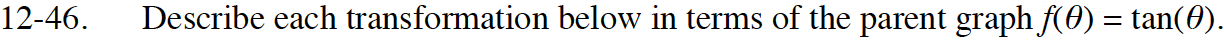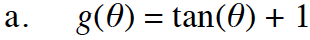Think of this function as f(x) = tan (θ) + 1.
What happens when you add one to a function? Consider a function like y = x2.

It is shifted up 1 unit.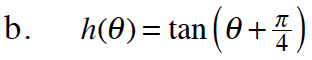What does it mean for the transformation to be inside the parentheses? How is this different from the transformation in part (a)? Again, consider how this works with other familiar functions.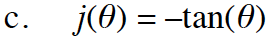How does a negative sign change a function?What does it mean to multiply the function by a number?

It is vertically stretched by a factor of 4.